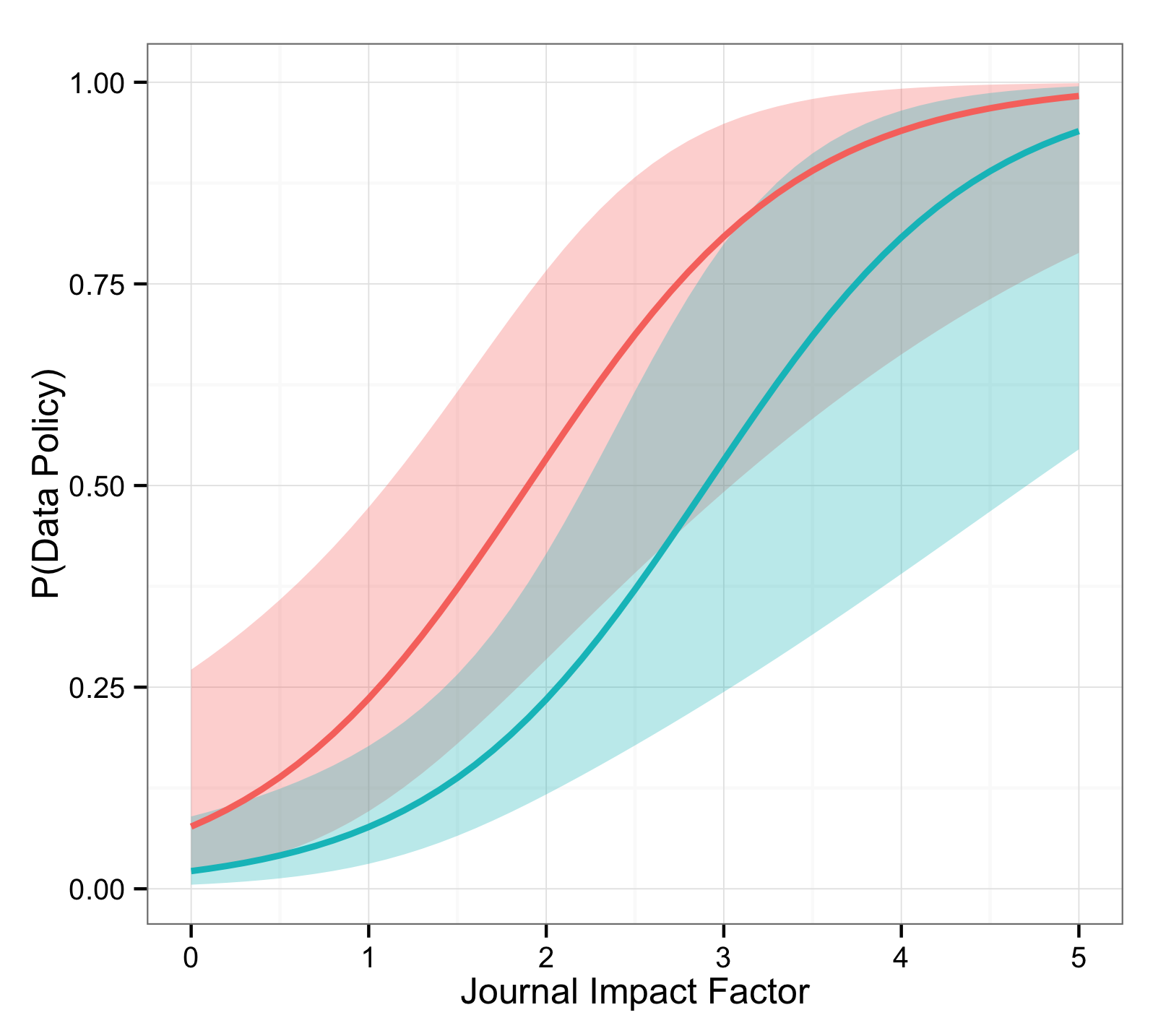# conjugateprior

## Which political science journals will have a data policy?

Making available replication materials for the research you do is A Good Thing. It's also work, and it's quite easy to never get around to. Certainly I claim no special virtue in this department so I am always happy when there's an institutional stick to prod my better nature in the right direction. One such institutional prod comes from academic journals and their data policies. If you have to give them your replication data before you they'll publish your paper, then you probably will. What sorts of journals have data policies?

In a recent paper looking at the relationship between Thomson ISI impact factor, audience, language, age, and issue frequency and the existence of a data policy, Gherghina and Katsanidou find that in a sample of political science journals the presence of a data policy mostly covaries with the journal's target audience - general or specialised - and with its impact factor. And to their eternal credit, the authors make their data available.

As one might hope, their analysis has already been replicated. (You should read this link for some useful discussion and citations). Ghergina and Katsanidou only present correlations, so we can dig a bit further with some modelling with their data. I do that below. But first, here's the headline.

The plot below represents the predicted probability of having a data policy for English-language general (red, higher) and specific (blueish-green, lower) journals as a function of their impact factor. The bands are two standard error pointwise confidence intervals, with approximately 95% coverage under the usual assumptions.Higher impact factors strongly predict higher probabilities of having a data policy, with general audience journals slightly more likely to have a data policy than specific journals.

Now it could be that better journals are better because they have a data policy, or that they try to signal their quality by having one, or that a data policy increases citation rates and thus the journal's impact factor, or that some other factors generate high impact factors and the desire for a data policy. That is, of course, not settled by anything we've done here. Nevertheless, knowing the impact factor and audience of a journal apparently tells you quite a lot about whether it's going to have a data policy.

### Trying it at Home

In the model represented in the plot I've assumed that only language (english or not), audience (general or specific) and impact.factor (from 0 to 5.22) matter for predicting a data policy.

#### Fitting a Model

Here's a straightforward logistic regression using my own version of the data

``````load('jpols.Rdata') ## jpols is my tidied-up version of the original data

glm.mod <- glm(data.policy ~ language + audience + impact.factor,
data=jpols, family=binomial)
``````

R's default listwise deletion procedure drops journals without impact factors when this model is fitted, which leaves 95 journals.

There's no need to dwell here on the model interpretation except perhaps to note that, in contrast to the correlations in the paper, the language of a journal no longer significantly predicts having a data policy. This is because of the tight connection between being in English, having an ISI ranking, and having a higher impact factor if ranked. (Similar arguments hold for the age of the journal, which I've simply left out.)

Perhaps the most interesting single quantity in this model is the predicted probability of a data policy for a unit increase in impact factor. A unit more impact makes it

``````logodds.impact <- coef(glm.mod)['impact.factor']
prop.increase <- exp(logodds.impact)-1 ## about 2.7
``````

nearly three times more likely that a journal has a data policy.

#### Constructing Data for Prediction

The figure requires predicted probabilities for English language journals with impact factors between zero and five for both general and specific audiences, so I make up that data:

``````imps <- seq(0,5,by=0.1) ## range of impact factors
eng.nd <- data.frame(language="english",
audience=rep(c("general", "specific"), each=length(imps)),
impact.factor=rep(imps, 2))
``````

and get predictions from the fitted model with standard errors.

``````preds <- predict(glm.mod, newdata=eng.nd, se.fit=TRUE)
``````

This gives standard errors for the link (a log odds) rather than the response (a probability) so I define the inverse link function invl to transform log odds into probabilities

``````invl <- function(x){ 1/(1+exp(-x)) }
``````

Next I make a data frame with everything needed for the plot: the audience, the impact factor, and the upper, lower and expected predicted probabilities suitably transformed

``````dd <- with(preds,
data.frame(audience=eng.nd\$audience,
impact=eng.nd\$impact.factor,
lower=invl(fit-2*se.fit),
pred=invl(fit),
upper=invl(fit+2*se.fit)) )
``````

An Aside: Why bother with this invl business? Why not ask directly for standard errors on the response by specifying type="response" in the predict function? Because this will return only one column of standard errors but with data where the predicted probabilities are close to zero or one, the correct predictive intervals will be strongly asymmetrical. For example, in this model the predicted probability for a general journal with impact factor 4 is 0.94 with a two standard error interval of [0.66, 0.99]. On the response scale, this would have been [0.82, 1.06] which is too high at one end and impossible at the other.

#### Plotting Predictive Probabilities

Plotting is relatively straightforward using the ggplot2 package

``````library(ggplot2) ## load package

ggplot(dd, aes(impact, pred, colour=audience)) +
geom_ribbon(aes(x=impact, ymin=lower, ymax=upper, fill=audience, linetype=NA),
alpha=0.3) +
geom_line(size=1) +
theme_bw() +
theme(legend.position="none") +
labs(x = "Impact Factor", y = "P(Data Policy)")
``````

And that's the final plot. I'm still getting my head around ggplot2 so there may be neater ways to do this.